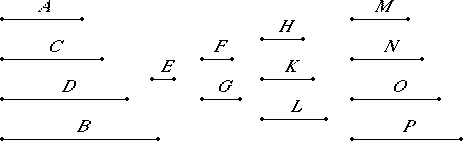# Proposition 9

If two numbers are relatively prime, and numbers fall between them in continued proportion, then, however many numbers fall between them in continued proportion, so many also fall between each of them and a unit in continued proportion.

Let A and B be two numbers relatively prime, and let C and D fall between them in continued proportion, and let the unit E be set out.

I say that, as many numbers fall between A and B in continued proportion as fall between either of the numbers A or B and the unit in continued proportion.VIII.2

Take two numbers F and G, the least that are in the ratio of A, C, D, and B, three numbers H, K, and L with the same property, and others more by one continually, until their multitude equals the multitude of A, C, D, and B. Let them be M, N, O, and P.

VIII.2,Cor

It is now manifest that F multiplied by itself makes H and multiplied by H makes M, while G multiplied by itself makes L and multiplied by L makes P.

VIII.1

And, since M, N, O, and P are the least of those which have the same ratio with F and G, and A, C, D, and B are also the least of those which have the same ratio with F and G, while the multitude of the numbers M, N, O, and P equals the multitude of the numbers A, C, D, and B, therefore M, N, O, and P equal A, C, D, and B respectively. Therefore M equals A, and P equals B.

Now, since F multiplied by itself makes H, therefore F measures H according to the units in F. But the unit E also measures F according to the units in it, therefore the unit E measures the number F the same number of times as F measures H.

VII.Def.20

Therefore the unit E is to the number F as F is to H.

Again, since F multiplied by H makes M, therefore H measures M according to the units in F. But the unit E also measures the number F according to the units in it, therefore the unit E measures the number F the same number of times as H measures M.

Therefore the unit E is to the number F as H is to M.

But it was also proved that the unit E is to the number F as F is to H, therefore the unit E is to the number F as F is to H, and as H is to M. But M equals A, therefore the unit E is to the number F as F is to H, and as H is to A. For the same reason also the unit E is to the number G as G is to L and as L is to B.

Therefore as many numbers fall between A and B in continued proportion as fall between each of the numbers A and B and the unit E in continued proportion.

Therefore, If two numbers are relatively prime, and numbers fall between them in continued proportion, then, however many numbers fall between them in continued proportion, so many also fall between each of them and a unit in continued proportion.

Q.E.D.

## Guide

The proposition says that if a and b are relatively prime, and they are the ends of a continued proportion with n terms, then a and b are each (n–1)st powers.

For example, a = 8 and b = 27 are relative prime and are the ends of the continued proportion, 8 : 12 : 18 : 27, therefore 8 and 27 are both cubes.

#### Outline of the proof

Suppose that relatively prime numbers a and b are the ends of a continued proportion with n terms, and that f : g is the ratio for the continued proportion in lowest terms. Then Euclid shows that a is the n 1st power of f, and b is the n 1st power of g. The argument is that the sequence

f n-1, f n-2g, f n-3g2, ..., fgn-2, gn-1

is in continued proportion with the correct ratio with relatively prime ends, so by VIII.1 they’re the same sequence.